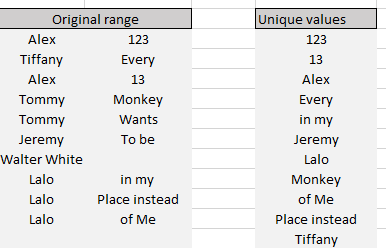One of the most useful functions in Excel is a function that shows unique values from a range. There is a native UNIQUE function but it doesn't do the job very well and isn't supported by older Excel versions, so let me present an alternative.

Here is a VBA code for Unique-values function (it goes with alphabetical sorting here):


'-------------------------------------------------------------------------
' Source: risksir.com
' Description: Deleting dublicates and forming the unique-values array
'-------------------------------------------------------------------------

Function UniqValues(SourceRange As Variant) As Variant
On Error Resume Next: Dim cell As Range, coll As New Collection, txt$$SourceArr = SourceRange ArrSize = UBound(SourceArr, 1) * UBound(SourceArr, 2) For Each cell In SourceRange.Cells txt$$ = Trim(cell): If Len(txt$$) Then coll.Add txt$$, txt\$
Next cell

ReDim uniqarr(1 To ArrSize, 1 To 1)
For i = 1 To coll.Count: uniqarr(i, 1) = coll(i): Next i

For i = coll.Count + 1 To ArrSize
uniqarr(i, 1) = ""
Next i

UniqValues = SortABC(uniqarr, 1)

End Function


'-------------------------------------------------------------------------
' Source: risksir.com
' Description: Alphabetical sorting by selected column
'-------------------------------------------------------------------------
Function SortABC(SourceRange As Variant, ByVal n As Integer) As Variant
' Sorting an array of SRTINGS by N-th column, sending empty strings to the end
SourceArr = SourceRange
If n > UBound(SourceArr, 2) Or n < LBound(SourceArr, 2) Then MsgBox "There's no " & n & " column in the array!", vbCritical: Exit Function
Dim Check As Boolean, iCount As Integer, jCount As Integer, nCount As Integer
ReDim tmpArr(UBound(SourceArr, 2)) As Variant
Do Until Check
Check = True
For iCount = LBound(SourceArr, 1) To UBound(SourceArr, 1) - 1
If ((UCase(CStr(SourceArr(iCount, n))) > UCase(CStr(SourceArr(iCount + 1, n))) Or CStr(SourceArr(iCount, n)) = "") And CStr(SourceArr(iCount + 1, n)) <> "") Then
For jCount = LBound(SourceArr, 2) To UBound(SourceArr, 2)
tmpArr(jCount) = SourceArr(iCount, jCount)
If SourceArr(iCount, jCount) = "" Then tmpArr(jCount) = ""
SourceArr(iCount, jCount) = SourceArr(iCount + 1, jCount)
SourceArr(iCount + 1, jCount) = tmpArr(jCount)
Check = False
Next
End If
Next
Loop
SortABC = SourceArr
End Function